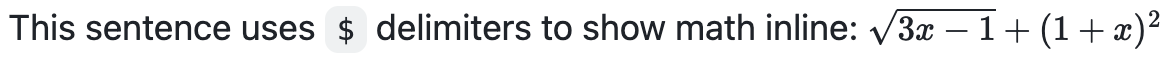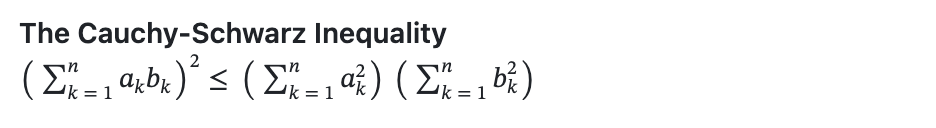# Writing mathematical expressions

Use Markdown to display mathematical expressions on GitHub.

To enable clear communication of mathematical expressions, GitHub Enterprise Server supports LaTeX formatted math within Markdown. For more information, see LaTeX/Mathematics in Wikibooks.

GitHub's math rendering capability uses MathJax; an open source, JavaScript-based display engine. MathJax supports a wide range of LaTeX macros, and several useful accessibility extensions. For more information, see the MathJax documentation and the MathJax Accessibility Extensions Documentation.

Mathematical expressions rendering is available in GitHub Issues, GitHub Discussions, pull requests, wikis, and Markdown files.

## Writing inline expressions

To include a math expression inline within your text, delimit the expression with dollar symbols $. This sentence uses $ delimiters to show math inline:  $\sqrt{3x-1}+(1+x)^2$## Writing expressions as blocks

To add a math expression as a block, start a new line and delimit the expression with two dollar symbols $$. **The Cauchy-Schwarz Inequality**$$\left( \sum_{k=1}^n a_k b_k \right)^2 \leq \left( \sum_{k=1}^n a_k^2 \right) \left( \sum_{k=1}^n b_k^2 \right)## Writing dollar signs in line with and within mathematical expressions

To display a dollar sign as a character in the same line as a mathematical expression, you need to escape the non-delimiter $ to ensure the line renders correctly. • Within a math expression, add a \ symbol before the explicit $.

This expression uses \$ to display a dollar sign:$\sqrt{\$4}$• Outside a math expression, but on the same line, use span tags around the explicit $. To split <span>$</span>100 in half, we calculate $100/2$